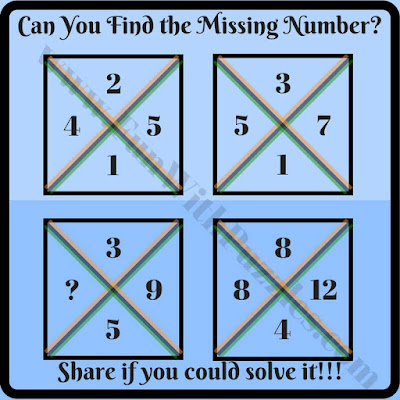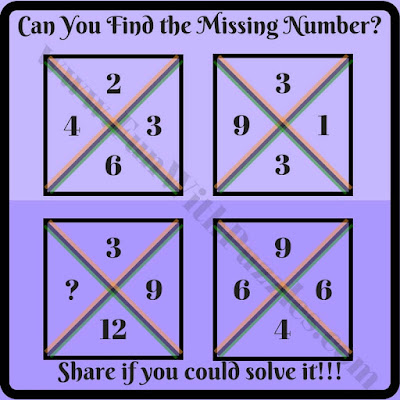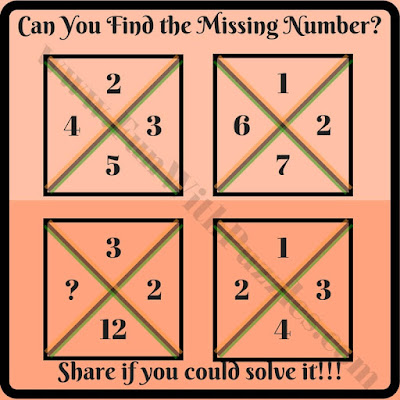Fun in Mathematics can be added with math brain teasers and riddles. One can find many different Math Brain Teasers and Riddles on the website. e.g. one can solve Circle Math puzzles in which one has to find the missing number in one of the circles out of four given circles. Similarly, there are Math Puzzles that are created with the shape of a square. Again in these puzzles, one has to find which number will replace the question mark in one of the squares. Similarly, we have sequence math puzzles for kids in which one has to find the next number in the sequence. Today we are publishing cross-squared math brain teasers that will add fun for school-going kids while they learn the basics of mathematics.

## How to Solve Maths Brain Teasers and Number Riddles

In these math brain teasers and riddles, four squares are given. Each square is divided by a cross into four parts and each of the parts is having one number. These numbers are related to each other by some mathematical operations. Find out this relationship and then find the missing number in one of the squares.

So are you ready to find the number which will replace the question mark? These math brain teases are easy for school-going kids. Teens and adults will find these riddles easy. Please post your answers to these puzzles in the comments.1. Maths Brain Teasers Number Puzzle Riddle2. Easy math square brain teaser riddle4. Simple Math Square Brain Teaser Riddle5. What will replace question mark brain teaser riddles?

## Answers to Missing Number Math Brain Teasers

Unknown said...

what is the answer for the 4th one

Fun With Puzzles said...